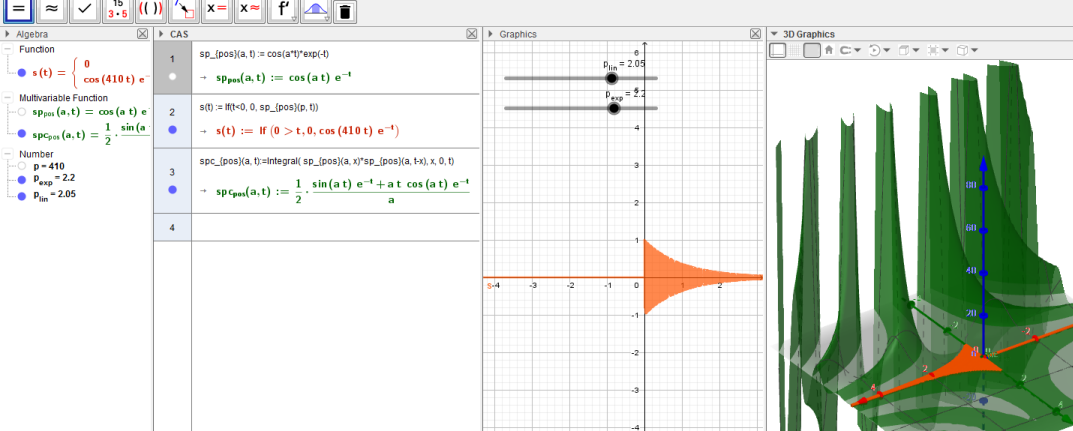# Limit should include the name of the variable

Falmir shared this idea 3 years ago
Declined

I'm busy with some calculation, where I use a cursor as parametre, and I had the surprise to see that when my cursor is zero, my function disappear, which was unexpected. Investigating my function (which results from an integral), i found out, that it contains something of the form : sin(a t)/a.

An easy solution would be to replace this by limite (sin(b t)/b, b->a) but the geogebra limite command does not allow to specify the variable name. the problem is that my function is in t and i need to have my limit calculated on a and not on t. And changing my variables names might have consequences on other default behaviours which favors t or x.1

in GG algebra the variable is the letter of the function. it is not necessary.

CAS has the Limit( <Expression>, <Variable>, <Value> ) command

saludos1

Hello,

Indeed, I was working in algebra and I didn't see that it was different in CAS than in GG algebra.

Now, I don't believe we can assume that Algebra functions only has one variable. This is especially needed when you want to compute a convolution produit or transforms like Fourier transforms. In GG classic my function do not appear on the graph when I write them in CAS.

Regards,1

CAS handles expresions, GG algebra handles expresions with graphic representation.

you can define f(x,y) but the limit[] comand does not handle two variables in algebra view because generally it has not a concrete representation.

if an expresion in CAS has representation then there is a empty dot at left, down the number of row. click on it and it will copied to algebra view, the dot becomes blue.1

Well.., it doesn't work : Here is a case (In GG classic)

let's create this function in CAS

1. sp_{pos}(a, t) = cos(a*t)*exp(-t)

then create a cursor in algebra view (this is a special exponential notation cursor)

1. p = p_{lin} 10^floor(p_{exp} + 0.5) 2^(floor(reste(3*p_{exp}+100.5, 3)) - 1)

then create this function in CAS (which perfectly graphs in algebra view)

1. s(t) = si(t<0, 0, sp_{pos}(p, t))

then let's skip this and compute my integral

1. spc_{pos}(a, t)=Intégrale( sp_{pos}(a, x)*sp_{pos}(a, t-x), x, 0, t)

It doesn't work if I don't copy sp_{pos}(a, x) in the algebra windows XD

( I wanted to try with GG6, but I never found how to paste my commands from my text file in it)1

perhaps if you put := in definition of functions.............see colonI did not copy anything

saludos1

Please see http://wiki.geogebra.org/fr... for basic info about entering and manipulating objects in CAS View.1

Ok, Thank you, I missed that point. I need to check again.

Is there a way to create my cursor as I was doing in the Algebra View ?1

you can create the cursosr in the algebra view and use it in CAS, like I did1

Hi,

Maybe this helps. Check the script in the button Setup.

I rather using 'x' and 'y' variables, but that is my preference. It helps me to define the functions and later define sliders in Algebra view that can be used with the functions.

Saludos1

Yes, that's what I was doing too. except that I was doing it all in algebra :-)1

Hi,

The only detail is that some commands are defined exclusively for CAS view or for Algebra view. So when you write a ggb script, you can not include a command from the CAS view. That is why I wrote in the script the command Integral[ <Function>, <Variable>, <Start x-Value>, <End x-Value> ] as a comment within the button Setup, since this works only in CAS view.

Saludos.